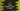# C# program to find random numbers in a range## How to create random number in range in C sharp:

To create a random number in C#, we can use Random class. It provides an easy way to generate random numbers in a given range.

We will use the below method for that:

``public virtual int Next (int minValue, int maxValue);``

It has two parameters: minValue and maxValue. minValue is the lower bound and maxValue is the upper bound of the random number returned by this method. The returned value is greater than or equal to minValue and less than maxValue. If both are equal, it will return minValue.

### Exception:

It will throw the below exception:

``ArgumentOutOfRangeException``

If minValue is greater than maxValue.

### Example program:

Let’s take a look at the below program:

``````using System;

public class Program
{
public static void Main()
{
Random r = new Random();
int randomNumber = r.Next(10,100);
Console.WriteLine(randomNumber);
}
}``````

If you run it, it will always print one random number in range 10 and 100.

### Example to find random number in range by taking user input of lower and upper bound:

We can also write one program that will take the lower and upper bound as input from the user and prints out one random number in that range. For example:

``````using System;

public class Program
{
private static int findRandom(int first, int second){
Random r = new Random();
return r.Next(first,second);
}

public static void Main()
{
Console.WriteLine("Enter the first number :");

Console.WriteLine("Enter the second number :");

Console.WriteLine("Random number between "+first+" and "+second+" is: "+ findRandom(first,second));

}
}``````

Here, we are taking the lower and upper bounds from the user. If you execute this program, it will print output as like below :

``````Enter the first number :
5
Enter the second number :
10
Random number between 5 and 10 is: 7``````

Creating a different method for all utility tasks is always a good choice :)